# Lesson 6: Unidirectional complex loads

In this lesson, we’ll delve into the fascinating world of unidirectional complex loads, exploring scenarios where materials experience a combination of forces and moments. These situations require a deep understanding of mechanics to analyze and design structures effectively.

1. Only tension (σ): Normal force + bending moment

Inclined bending

Excentric tension/compression

1. Only shear (𝜏): Shearing force + twist

Does not exist!

1 INCLINED BENDING

Definition: The moment vector is not parallel to any of the prime moment of inertia axes of the cross-section.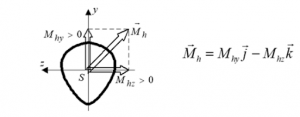• Calculation: Simply the superposition of two straight bendings.
• Stress State: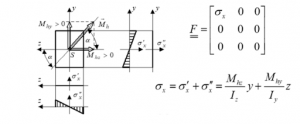2. Excentric tension/compression

• Definition: The load is eccentric when the resultant of the force system acting on the rod is parallel to the axis of the rod but not in the centroid of the cross-section.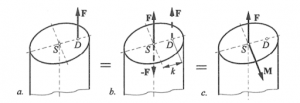• Solution: Reduction of the eccentric load to the centroid. Result: moment and force.
• Calculation: superposition of tensile stress from tension and bending.

1 INCLINED BENDING

• Definition: The moment vector is not parallel to any of the prime moment of inertia axes of the cross-section.
• Calculation: Simply the superposition of two straight bendings.
• Stress State:
•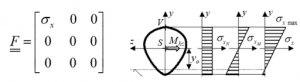• Calculation: superposition of tensile stress from tension and bending.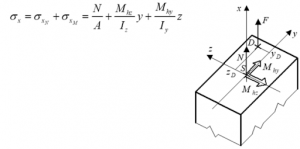Unidirectional complex loads challenge engineers and designers to consider the interplay between forces and moments in materials. A strong grasp of mechanics, stress analysis, and structural design is essential when dealing with such scenarios. This knowledge empowers professionals to create safe and efficient structures, ensuring they can withstand the demands of real-world applications.

With this understanding of unidirectional complex loads, you’ll be better equipped to tackle complex engineering challenges and make informed decisions in various industries, from civil engineering to aerospace.